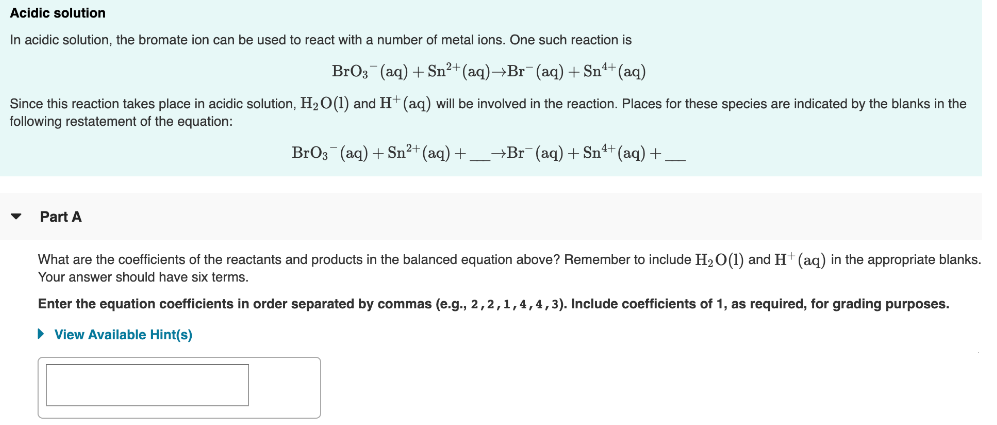# In acidic solution, the bromate ion can be used to react with a number of metal ions. One such reaction is BrO3^-(aq) + Sn^2+(aq) → Br^-(aq) + Sb^4+(aq) Since this reaction takes place in acidic solution, H2O(l) and H+ (aq) will be involved in the reaction. Places for these species are indicated by the blanks in the following restatement of the equation. BrO3^-(aq) +Sn^2+(aq) + ___ → Br^-(aq) + Sn^4+(aq) ___ What are the coefficients of the reactants and products in the balanced equation above? Remember to include H2O(l) and H+(aq) in the appropriate blanks. Your answer should have six terms. Enter the equation coefficients in order separated by commas (e.g., 2, 2, 1, 4, 4, 3). Include coefficients of 1, as required, for grading purposes.